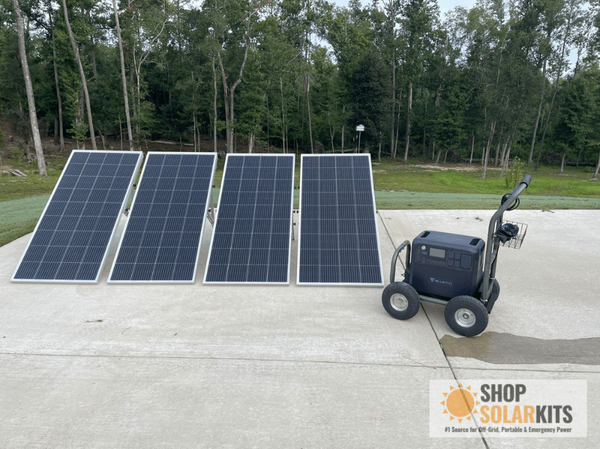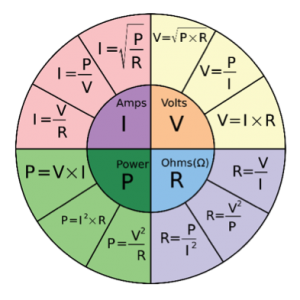# Watt Density Calculator

A watt density calculator allows you to better understand the efficiency and lifespan of your heating element. Rather than having to spend time on complicated calculations, you can simply enter the total wattage and the area in inches squared into an easy formula to find out your watt density.

## What Is Watt Density?

When we talk about watt density, we are referring to heating elements. This includes both coil heaters and surface heaters.

Watt density is the wattage of a heating element in relation to its size, and could also be referred to as the heat flux. It is the most important aspect of a heater’s output and efficiency.

Watt density is important in understanding the operating temperature of a heater under certain conditions. If you know the watt density of your heating element then you can get a better sense of if it is meeting your needs.

Understanding the watt density of your heating element also allows you to understand the operating temperature of your heating element. This can be especially useful if your heating element is powered by a solar generator, like the Bluetti AC200 Max solar generator.You can make sure to match the watt density of your heating element with the required output for your home and the specs of your solar generator. This also applies if you are using conventional electricity.

With a solar generator, you can also use a DC watts to AC watts calculator to understand the efficiency of your generator.

In the U.S., watt density is normally expressed in watts per square inch (wpsi). This refers to the wattage of each square inch of your heating element. Certain water heaters have higher or lower watt density.

However, heating elements with lower watt density are normally larger to make up the difference.

## What Is A Watt Density Calculator?

A watt density calculator allows you to understand the wattage and determine the output of your heating element. You can insert your variables into a formula that will tell you the watt density.

A watt density calculator is different from a watts-to-VA calculator, or a watts-to-amp hours calculator

With a watt density calculator, you can then use this information to understand how to make your heating element as efficient as it possibly can be. Using the formula allows you to get a precise sense of your watt density without having to work out each individual component.

Watt density is also very useful when it comes to choosing a heating element and deciding between different options. You can find one that best suits your needs. With a higher watt density, you can opt for a smaller heating element.Watt density is directly correlated to transfer efficiency and also has some bearing on the lifespan of your heating element.

At its most basic, the formula for calculating watt density is power divided by surface area. This means the wattage divides by the area of your heating element in inches squared, giving you wpsi.

## Why Is a Watt Density Calculator Important?

For an optimal experience and lifespan, you want your wpsi to be no more than 35 for a ceramic band heater. You need to balance the space you have with the output you need. In most cases, you can find a lower wpsi equivalent to a heating element with a higher watt density.

The key difference is that heating elements with a lower watt density have a lower operating temperature compared to those with a higher watt density.

Because of this lower operating temperature, heating elements with a lower wpsi can last longer.

Another important part of understanding your heating element is being able to convert watts to watt-hours

There are many factors when it comes to understanding exactly how your heating element works, but watt density is an important place to start.

You need to take the total wattage of your heating element and divide it by the area in inches to get the wpsi.

For example, a 2” by 2” heating element with a wattage of 100 W would have a watt density of 25wpsi. You get this by going 100/2x2. This is within a good range for a ceramic band heater.## Conclusion

Now that we’ve covered the basics, we’re sure you’ll be able to find the perfect heating equipment for your needs.

However, solar panel knowledge doesn’t stop there. There’s a long list of other calculations you should familiarise yourself with, and we’ve got plenty of useful resources for you to use. You can check out our watts to mAh calculator or even our Watt’s law calculator for all your electrical needs.

Did You Find Our Blog Helpful? Then Consider Checking: